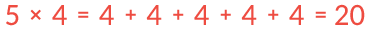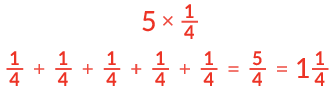# Set 5 Multiplying & Dividing FractionsYou know thatYou can use that same strategy when you multiply a fraction and a whole number.

5 x ¼ can be thought of as adding ¼ five times.How is multiplying fractions different than multiplying whole numbers?